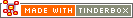min(set)Operator Type: Set maths Operator Scope of Action: Set

The min() operator returns the largest item in a set.

Both the operators max() and min() use lexical comparison in most cases, but numeric comparison if the context is numeric, e.g.:

`Width=min("100;2;70")`

Since "Width" is numeric, min() will be return 2.

`Name=min("100;2;70")`

Since "Name" is a string, mix() will return 100.

If you don't have a set, create one on the fly:

`MyMin = min(collect_if(all,\$MyNum>0,\$MyNum))`

A 'lexical sort' is a case-insensitive sort (Aardvark,aarvark,ABLE) and for numbers "2;70;100;" would sort in order "100;2;70" (i.e. ordered on first number first - a number 'computer sort' )

 Up: Full Operator List Previous: max(set) Next: mod(argumentA,argumentB)

[Last updated: 14 Dec 2009, using v5.0]